In :
import scipy.signal

def plotspec(x, Ts):
fig = figure()
ax1.plot(x)

q = fft.fft(x)
ax2.plot(fft.fftfreq(len(x), Ts), abs(q))

time = 0.3
Ts = 1.0/10000.0
t = linspace(Ts, time, time/Ts-1)
lent = len(t)
fc = 1000.0
c = cos(2*pi*fc*t)

fm = 20.0

w = 5.0/lent*(linspace(1, lent, lent))+cos(2*pi*fm*t)

v = c*w
gamma = 0
phi = 0
c2 = cos(2*pi*(fc+gamma)*t+phi)

x = v*c2
fbe = [0, 0.05, 0.1, 0.5]
damps = [1,0]
fl = 100
b = scipy.signal.remez(fl,fbe,damps)
m = 2*scipy.signal.lfilter(b,1,x)

plot(w)
figure()
plot(v)
figure()
plot(m)

Out:
[<matplotlib.lines.Line2D at 0x1112566d0>]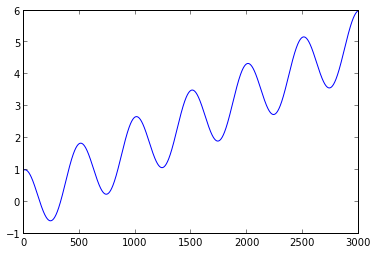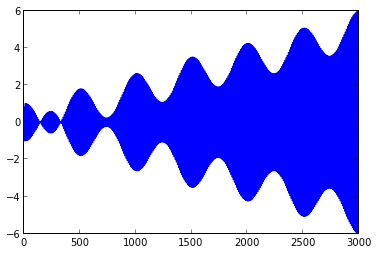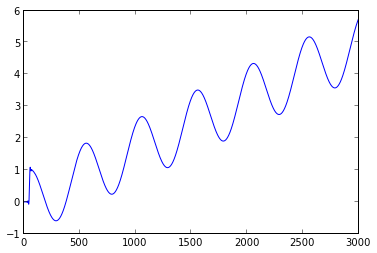5.5. Plot the spectra of w,v,x, and m(t).

In :
def modAM(w, fc, phi, Ts):
time = Ts*len(w)
t = linspace(0, time, len(w))

c = cos(2*pi*fc*t+phi)

v = c*w

return v

def demodAM(v, fc, Ts):
time = Ts*len(w)
t = linspace(0, time, len(w))

c2 = cos(2*pi*fc*t+phi)

x = v * c2

fbe = [0, 0.05, 0.1, 0.5]
damps = [1,0]
fl = 100

b = scipy.signal.remez(fl, fbe, damps)

m = 2*scipy.signal.lfilter(b,1,x)

return m

Ts = 1.0/10000.0

w = 5.0/lent*(linspace(1, lent, lent))+cos(2*pi*fm*t)
v = modAM(w, 1000.0, 0.0, Ts)

m = demodAM(v, 1000.0, Ts)

plotspec(w, Ts)
plotspec(v, Ts)
plotspec(m, Ts)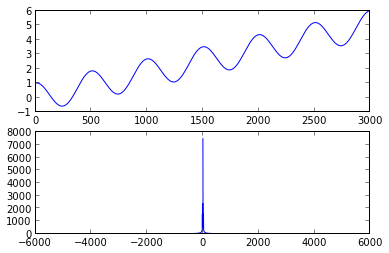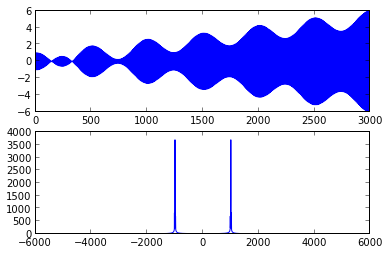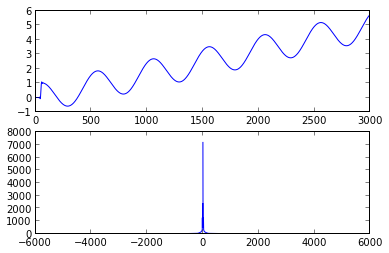5.6. Try different phase offsets. How well does the recovered message match? What does ths spectrum of m look like?

In :
Ts = 1.0/10000.0

w = 5.0/lent*(linspace(1, lent, lent))+cos(2*pi*fm*t)

for phi in [-pi, -pi/2, -pi/3, -pi/6, 0, pi/6, pi/3, pi/2, pi]:
v = modAM(w, 1000.0, phi, Ts)
m = demodAM(v, 1000.0, Ts)

plotspec(m, Ts)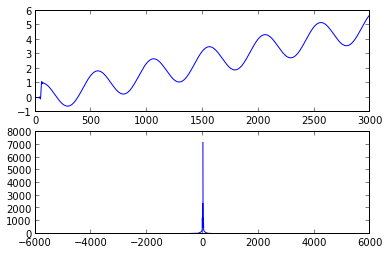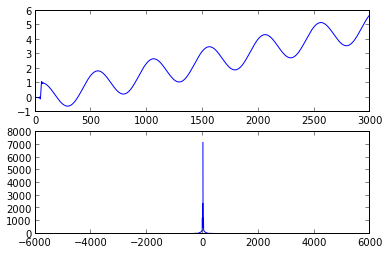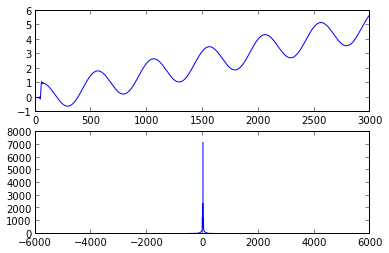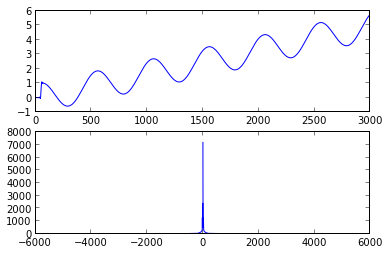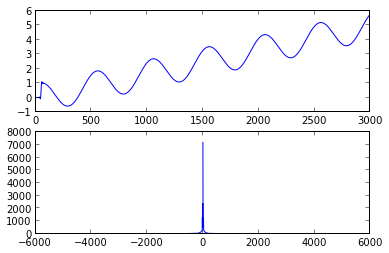5.7. Try different frequency offsets: gamma = [0.01, 0.1, 1.0, 10]. How well does the recovery match?

In :
Ts = 1.0/10000.0

w = 5.0/lent*(linspace(1, lent, lent))+cos(2*pi*fm*t)

for gamma in [0.01, 0.1, 1.0, 10.0]:
v = modAM(w, 1000.0+gamma, 0.0, Ts)
m = demodAM(v, 1000.0, Ts)

plotspec(m, Ts)
xlim((-100,100))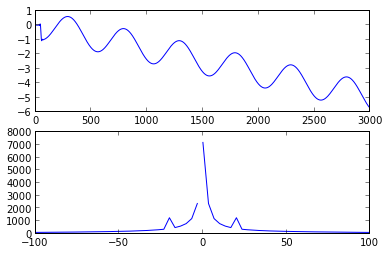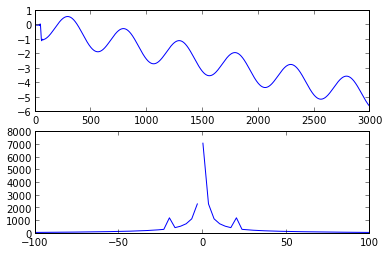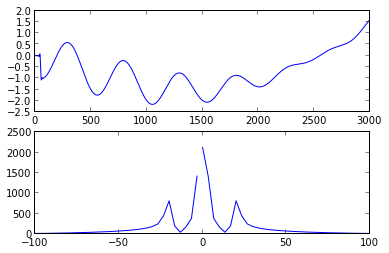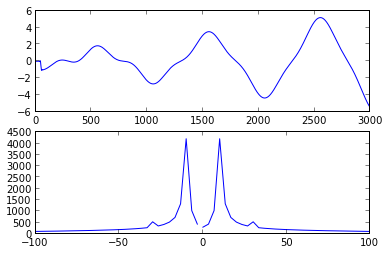5.9. Create a routine to implement the square-law mixing modulator of Figure 5.8.

In []:
def modSquareLaw(w, a0, fc, bw, Ts):
t = linspace(0, len(w)/Ts, Ts)
c = a0*cos(2*pi*fc*t)

x = w+c
y = x*x

Fs = 1.0/Ts

bpe = [0, (fc-bw-0.1*bw)/Fs, (fc-bw)/Fs, (fc+bw)/Fs, (fc+bw+0.1*bw)/Fs, 0.5]
damps = [0,1,0]

b = scipy.signal.remez(100, bpe, damps)